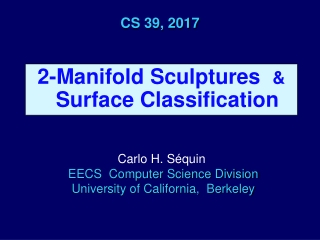DownloadDownload PresentationCS 39, 2017

# CS 39, 2017

Download Presentation## CS 39, 2017

- - - - - - - - - - - - - - - - - - - - - - - - - - - E N D - - - - - - - - - - - - - - - - - - - - - - - - - - -
##### Presentation Transcript

1. CS 39, 2017 2-Manifold Sculptures &Surface Classification Carlo H. Séquin EECS Computer Science Division University of California, Berkeley

2. How Mathematicians May Look at Sculptures by Eva Hildand Charles Perry 2-Manifold Sculptures &Surface Classification

3. (Approximate) 2-Manifolds Structures made out of thin sheets of material, Paper, sheet metal … -- examples: a paper cone, coke can …

4. Mathematical 2-Manifolds Mathematicians abstract this to the point where it is an infinitely thin sheet of “math substance.” Interior: disk-like neighborhoods. 2-Manifolds my have punctures (holes with sharp borders) Along those borders: half-disk neighborhoods.

5. “Hollow” by Eva Hild, Varberg, 2006

6. Eva Hild An large collection of ceramic creations & metal sculptures

7. Topological Analysis Surface Classification Theorem: • All 2-manifolds embedded in Euclidean 3-spacecan be characterized by 3 parameters: • Number of borders, b: # of 1D rim-lines; • Orientability, σ: single- / double- sided; • Genus, g, or: Euler Characteristic, χ,specifying “connectivity” . . .

8. Determining the Number of Borders Run along a rim-line until you come back to the starting point; count the number of separate loops. Here, there are 4 borders:

9. Determining the Surface Orientability A double-sided surface • Flood-fill_paint the surface without stepping across rim. • If whole surface is painted, it is a single-sided surface(“non-orientable”). • If only half is painted,it is a two-sided surface(“orientable”).The other side can then be painted a different color.

10. Determining Surface Orientability (2) A shortcut:If you can find a path to get from “one side” to “the other” without stepping across a rim, it is a single-sided surface.

11. Determining the Genus of a 2-Manifold Closed surfaces (handle-bodies) Surfaces with borders (disks with punctures) genus 0 genus 2 All: genus 0 The number of independent closed-loop cuts that can be made on a surface, while leaving all its pieces connected to one another. genus 4

12. Determining the Euler Characteristic From this: • Genus = 2 – χ – b for non-orientable surfaces; • Genus = (2 – χ – b)/2 for double-sided surfaces. Sometimes a simpler approach: Find χ • χ = V – E + F = Euler Characteristic • How many cuts(rim-to-rim) are neededto obtain a single connected disk? • Disk: χ = 1; do the inverse process:every cut re-join lowers χ by 1; thus “Tetra” ribbon frame: χ = –2

13. Determining the Euler Characteristicof Tubular Structures Cutting a tube (“circular” cut) does not change χ: • χ = V – E + F = Euler Characteristic • How many cuts to obtain a tree-like connected graph? • A tree-like tubular graph structure has genus = 0 It is a “sphere” with n “openings” = n punctures. • Each cut done adds +1 to the genus of the structure E.g., a tubular Tetrahedral frame has genus = 3 • If the structure was single-sided (Klein bottle),multiply by 2 the calculated genus

14. Costa_in_CubeCarlo Séquin, 2004, bronze Double-sided (orientable) Number of borders b = 3 Euler characteristic χ = –5 Genus g = (2 – χ – b)/2 = 2 Independent cutting lines: 2

15. “Endless Ribbon”Max Bill, 1953, stone • Single-sided (non-orientable) • Number of borders b = 1 • E.C. χ= 2–3+1= 0 • Genus g= 2 – χ – b= 1 • Independent cutting lines: 1

16. “Möbius Shell”Brent Collins, 1993, wood • Single-sided (non-orientable) • Number of borders b = 2 (Y,R) • Euler characteristic χ = –1 • Genus g= 2 – χ – b= 1 • Independent cutting lines: 1blueorgreen–but not both,they would intersect!